# Dynamic modelling

(Redirected from DynamicModelling)
NLA
QRSlv
CMSlv
IPSlv
NLP
CONOPT
IPOPT
TRON
MINOS
Opt
NGSlv
DAE/ODE
IDA
LSODE
DOPRI5
LA
Linsolqr
Linsol
LP
MakeMPS
Logic
LRSlv

Dynamic modelling in ASCEND is where you solve a system of ordinary differential equations (ODEs) or differential/algebraic equations for the time-varying values of variables in the model over a certain interval, given the initial conditions of the model. We also refer to this as integration. Dynamic modelling can be done within the ASCEND language providing the relationship between variables and their derivatives can be established.

ODE solvers of the LSODE and Runge-Kutta class solve problems that can be written: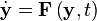$\dot{\mathbf{y}} = \mathbf{F} \left(\mathbf{y},t \right)$

by projection schemes for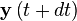$\mathbf{y}\left(t+dt\right)$ which require solving some set of equations based on Jacobian matrix of partial derivatives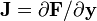$\mathbf{J} = \partial \mathbf{F} / \partial \mathbf{y}$ (also referred to as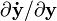$\partial \mathbf{\dot{y}}/\partial \mathbf{y}$) being nonsingular. Variants of ODE solvers may allow convergence with limited singularity in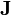$\mathbf{J}$ by using singular value decomposition (SVD) and assumptions about the properties of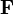$\mathbf{F}$, but this is not general or robust enough to be used in arbitrary models created with ASCEND.

The ASCEND representation of differential equations allows the more general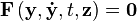$\mathbf{F}\left(\mathbf{y},\mathbf{\dot{y}},t,\mathbf{z}\right)=\mathbf{0}$

where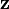$\mathbf{z}$ are apparently non-differential variables (variables that appear in the equations without a corresponding derivative), and$t$ is the (non-differential) independent (time) variable. Considering all variables$\mathbf{y}$,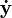$\mathbf{\dot{y}}$,$t$,$\mathbf{z}$ as algebraic, then the matrix$\mathbf{J}$ can be calculated analytically (within some tolerance) if the following is true:

With y fixed, t fixed, and as many z fixed as necessary, a well posed (square, nonsingular) nonlinear problem is obtainable.

This form is precisely the matrix needed at the initial condition, normally. So for each "function evaluation" of LSODE, we solve the nonlinear problem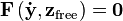$\mathbf{F} \left( \mathbf{\dot{y}}, \mathbf{z}_\mathrm{free}\right)=\mathbf{0}$ such that st.$\mathbf{y}$,$t$,$\mathbf{y}$,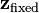$\mathbf{z}_\mathrm{fixed}$ are all fixed

and for each$\mathbf{J}$ needed we take the Jacobian of$\mathbf{F}$ and solve to get a column of sensitivity data. (In principle this can be accelerated by the method of Curtis, Powell, and Reid(citation needed), but that so rarely applies we didn't bother).

With the above technique, it may turn out that$\mathbf{J}$ is singular, even under SVD. This is the usual first indicator of a numerical index problem.

It turns out that in many cases the index problem is even visible structurally in QRSlv -- meaning there is no feasible way to form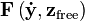$\mathbf{F}\left(\mathbf{\dot{y}}, \mathbf{z}_\mathrm{free}\right)$ by computing all the variables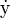$\mathrm{\dot{y}}$ expected and fixing all the variables$\mathbf{y}$ expected. In this case either the problem is badly posed or a proper ODE problem can be recovered by moving some pair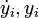$\dot{y}_i, y_i$ into the set$\mathbf{z}$ and perhaps adding extra constraints obtainable by differentiation of a subset of$\mathbf{F}$.

DASSL and IDA work around low index problems by solving all model and stepping simultaneously, but for problems typical in chemical engineering (setting pressure specifications on multistage operations like distillation) DASSL's technique is not enough for reliable solution even if SVD is used under the covers.

So basically, it's great to use DASSL for performance reasons over LSODE and the like. If the reason for using DASSL, IDA and the like is because LSODE fails, then the modeling approach should be carefully reevaluated.

If the reason for using DASSL/IDA is that they are better at finding initial conditions than LSODE (which has no such functionality), then again the main strength of ASCEND's primary solvers (getting solutions to hard nonlinear problems) is being overlooked. Ben Allan has heard many times of people using dassl as simply a nonlinear solver and integrating nothing at all.

Finally, now that memory is essentially free, boundary value problem (BVP) solution techniques should be of much greater interest than historically, and it just happens that ASCEND has a very nice BVP library: models/bvp.a4l

## Syntax for dynamic modelling

The current method for specifying derivatives is by including the ivpsystem.a4l library. Full details are given in Design and Use of Dynamic Modeling in ASCEND IV (see our publications page).

REQUIRE "ivpsystem.a4l";


at the start of your model, then creating a special method

METHOD ode_init;
dx_dt.ode_id := 1;
x.ode_id := 1; (* ie these variables are related with ID '1' *)

dx_dt.ode_type := 2; (* ie 'this is a derivative variable' *)
x.ode_type := 1; (* ie 'this is a differential variable *)

t.ode_type:= -1; (* 'tag' the independent variable *)
END ode_init;


which must be run before sending your model to either IDA or LSODE (or a future solver...) for integration.

## New syntax

For a full discussion on this topic, see Improved DAE syntax

Dante Stroe recently added support for LINK semantics that allows this fairly painful syntax to be improved, however the new code is not yet incorporated into the ASCEND 'trunk' (see svn info). Based on the LINK structure the following syntax can now be used to define derivative chains, for example:

INDEPENDENT t;
DER(dx_dt,x)


This syntax can be used in either the declarative part of the model, either in the Methods section.

• The INDEPENDENT keyword adds an independent variable to the solver. While it is possible to store multiple independent variables in the solver data structures, the user is currently limited to only one independent variable, since there is no implementation of partial derivatives. (Implementation-wise, a LINK entry with the 'indepenendent' key and the independent variable itself is created)
• The DER keyword is used to declare the derivative chains in terms of the declared independent variable. The parameters of DER are the differential variables in decreasing order (ode_type in the previous syntax). The derivative chains declared in DER can be arbitrarily long, however the solver does not handle more than 2nd order derivatives. (Implementation-wise, a LINK entry with the 'ode' key and the differential variable is created)

The following models using the aforementioned syntax have been added:

An inherent weakness of the syntax separating INDEPENDENT from der, as given above, is that in a hierarchically or peerwise composed model, multiple definitions of the same independent variable are possible and may conflict (or this may be by modeler choice, with multiple integrals within an overall model being computed by an IVP solver in different phases of the same simulation process). Several workarounds are possible. All rely on there being a community, or at least a library, standard way of doing things at the modeling level and an IVP solver understanding the convention.

• Syntactically: change the LINK-equivalent usage to be created from DER(dxdt,x,t) rather than DER(dxdt,x(*solver search for independent and insert here*)). Verbose but unambiguous. Solver pre-analysis still must verify that there is only one atom instance (t) that is the target of all DER definitions, even if that instance has multiple names and declarations that may have been merged.
• Typing an ATOM type to enforce that there exactly 1 independent: UNIVERSAL ATOM time_domain REFINES time; All instances of time_domain will automatically be the same. IVP Solvers can examine atom types and identify independent variables as those of type *_domain, eliminating the INDEPENDENT declaration. This solution actually allows multiple domains, and a parameter to an IVP analysis routines is "which domain type" is the independent variable. This would allow switching integrals over space(1-d) and integrals over time in the same model, an interesting capability in Low-Order PDEs. It might also allow interesting analyses in Fourier spaces.

Current DER style doesn't scale to 2 simultaneous independent dimensions: INDEPENDENT x,y; DER(pdUxy,U). In a simultaneous 2-d world, the universal atom approach fails. One can (and probably JP has) argue that PDE models must explicitly represent the grid at the ascend language modeling level, as in the BVP library. We note, however, that NO current production PDE-oriented DSL actually works this way: they all make declarations based on sets and boundaries with arbitrary external definition whose consistency is checked by the solver.